# AP Chemistry : Photoelectron Spectroscopy

## Example Questions

### Example Question #1 : Photoelectron Spectroscopy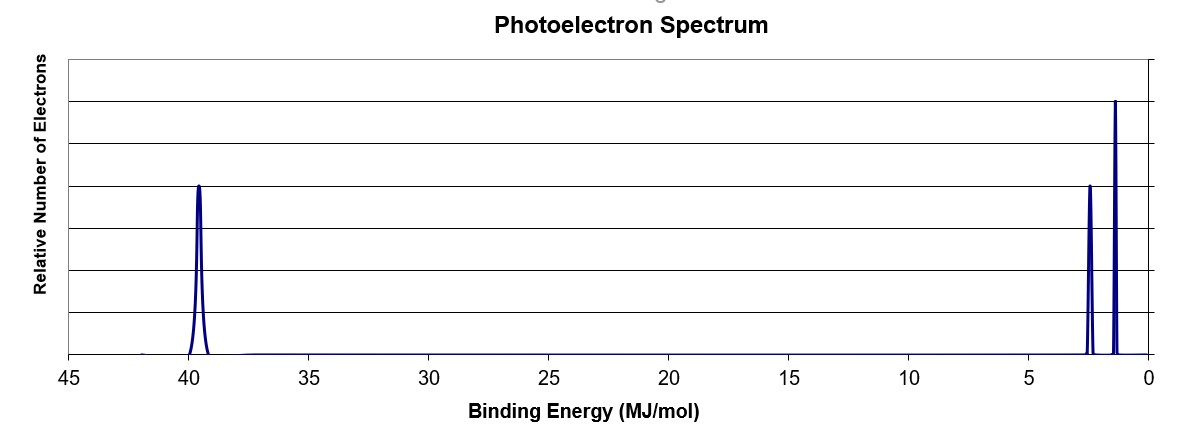Using the PES spectra above, what element is illustrated?

Na

N

Ne

C

Li

N

Explanation:

The peak at ~ 40 MJ binding energy corresponds to the 1s orbital.  Since there are peaks at lower binding energy, it is implied that this orbital is filled (by 2 electrons).  There are two peaks at lower binding energy corresponding to the 2s and 2p orbitals, respectively.  The 2s peak has the same intensity as the 1s peak indicating it has the same number of electrons (2).  The 2p peak is 3/2 as tall as the 2s peak and indicates that indicates it has 3 electrons in the orbital.  The electron configuration of 1s22s22p3 makes the element N.

### Example Question #1 : Photoelectron Spectroscopy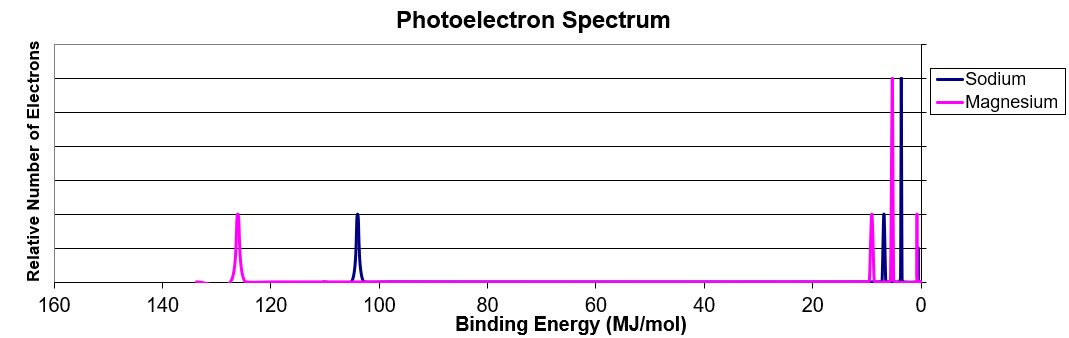Using the PES spectra above, what answer explains the differences in the position and intensity of the 3s peaks between Na and Mg?

Na experiences a greater shielding effect from the 2s and 1s electrons making the 3s electrons easier to remove.

Na experiences a larger effective nuclear charge on the 3s electrons and are thus harder to remove.

Mg is larger in size making the electrons in the 3s orbital farther from the nucleus and thus easier to remove

None of the above

Mg experiences a larger effective nuclear charge and has more electrons in the 3s orbital.

Mg experiences a larger effective nuclear charge and has more electrons in the 3s orbital.

Explanation:

Mg has a Z=12 while Na is Z=11.  The increased number of protons in Mg gives rise to a greater effective nuclear charge.  This greater effective nuclear charge binds the electrons in their respective orbitals more tightly than an element with a lower effective nuclear charge.  The peak height of Mg is 2x that of Na for the 3s orbital because it has twice the electrons present.

### Example Question #1 : Photoelectron Spectroscopy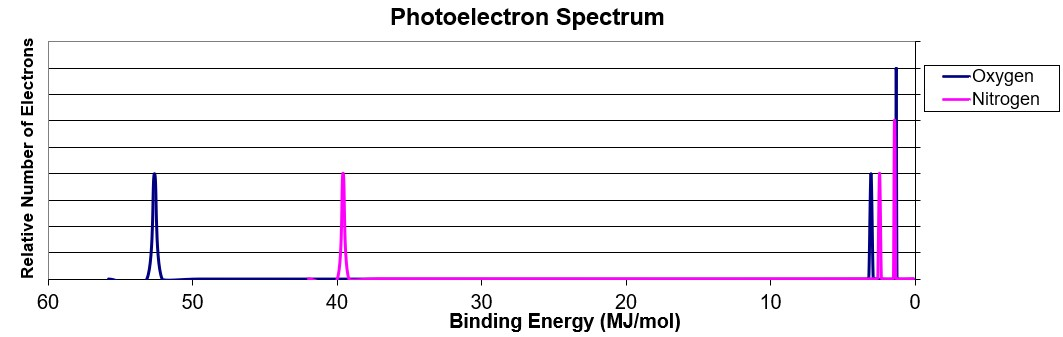Using the spectra above, answer the following:  Why is the oxygen 1s electrons further to the right than the nitrogen 1s orbital?

Nitrogen has 3 p-electrons and are thus unpaired leading to lower electron-electron repulsions.

The effective nuclear charge on oxygen is greater than that of nitrogen.

Nitrogen is larger than oxygen thus making the 1s electrons easier to remove.

There is a greater degree of electron shielding occurring in the oxygen 2p orbital.

Oxygen has more electrons and thus a greater amount of electron-electron repulsion present in the atom.

The effective nuclear charge on oxygen is greater than that of nitrogen.

Explanation:

Oxygen has a Z=8 vs nitrogen’s Z=7. This greater effective nuclear charge binds the electrons in their respective orbitals more tightly than an element with a lower effective nuclear charge.

### Example Question #1 : Photoelectron Spectroscopy

Using the spectra above, answer the following:  What is the electron configuration of the element shown above?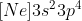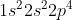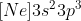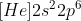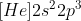Explanation:

The binding energy at ~ 53 Mj represents the 1s orbital.  With other peaks present, this 1s orbital is fully occupied by 2 electrons.  The peaks at lower energy would correspond to the 2s and 2p, respectively.  Since the 2p peak is twice the intensity of the 2s or 1s peak, it has twice the electrons (4).  This gives the electron configuration 1s22s22p4.

### Example Question #2 : Atomic Structure And Properties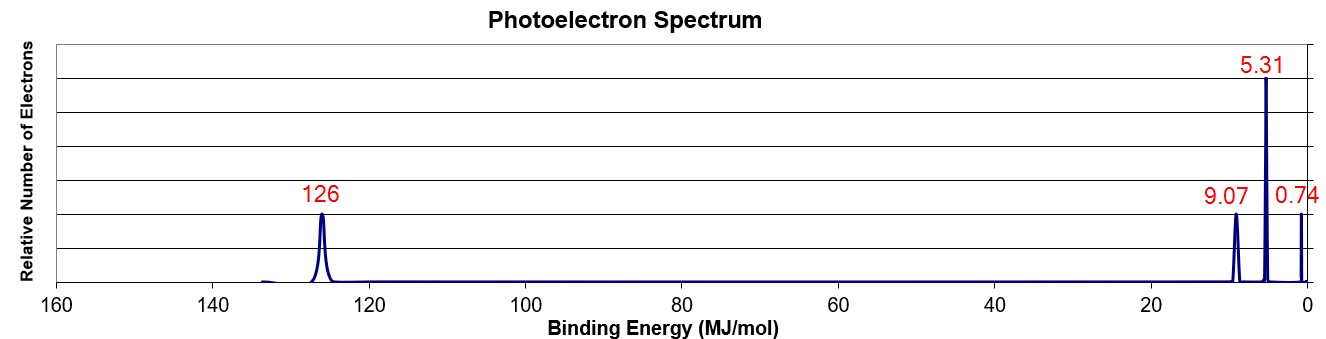Using the spectra above, answer the following:  What is the wavelength required, in m, to remove a valence electron from the element shown above?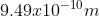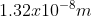None of the above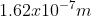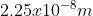Explanation:

Step 1.  Convert the Binding Energy from MJ/mol to J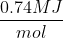x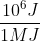x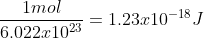Step 2: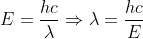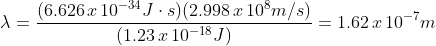### All AP Chemistry Resources